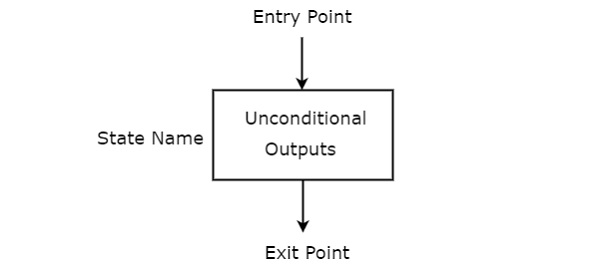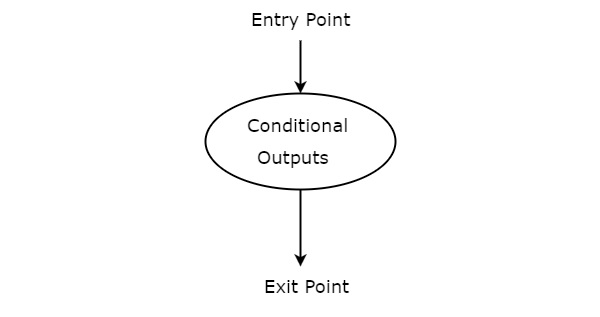# Digital Circuits - Algorithmic State Machines

Every digital system can be partitioned into two parts. Those are data path (digital) circuits and control circuits. Data path circuits perform the functions such as storing of binary information (data) and transfer of data from one system to the other system. Whereas, control circuits determine the flow of operations of digital circuits.

It is difficult to describe the behavior of large state machines using state diagrams. To overcome this difficulty, Algorithmic State Machine (ASM) charts can be used. ASM charts are similar to flow charts. They are used to represent the flow of tasks to be performed by data path circuits and control circuits.

## Basic Components of ASM charts

Following are the three basic components of ASM charts.

• State box
• Decision box
• Conditional output box

### State box

State box is represented in rectangular shape. Each state box represents one state of the sequential circuit. The symbol of state box is shown in the following figure.It is having one entry point and one exit point. Name of the state is placed to the left of state box. The unconditional outputs corresponding to that state can be placed inside state box. Moore state machine outputs can also be placed inside state box.

### Decision box

Decision box is represented in diamond shape. The symbol of decision box is shown in the following figure.It is having one entry point and two exit paths. The inputs or Boolean expressions can be placed inside the decision box, which are to be checked whether they are true or false. If the condition is true, then it will prefer path1. Otherwise, it will prefer path2.

### Conditional output box

Conditional output box is represented in oval shape. The symbol of conditional output box is shown in the following figure.It is also having one entry point and one exit point similar to state box. The conditional outputs can be placed inside state box. In general, Mealy state machine outputs are represented inside conditional output box. So, based on the requirement, we can use the above components properly for drawing ASM charts.